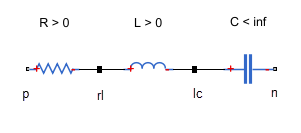## Component Variants — Series RLC Branch

The following example shows a series RLC component that implements a single resistor, inductor, or capacitor, or a series combination of these elements. The component uses conditional sections to implement the control logic.

```import foundation.electrical.electrical; % electrical domain class definition import foundation.electrical.elements.*; % electrical elements component SeriesRLC nodes p = electrical; % +:left n = electrical; % -:right end nodes(Access=protected, ExternalAccess=none) rl = electrical; % internal node between r and l lc = electrical; % internal node between l and c end parameters R = {0, 'Ohm'}; L = {0, 'H'}; C = {inf, 'F'}; end if R > 0 components r = resistor(R=R); end connections connect(p, r.p); connect(r.n, rl); end else connections connect(p, rl); % short circuit p--rl end end if L > 0 components l = inductor(l=L); end connections connect(rl, l.p); connect(l.n, lc); end else connections connect(rl, lc); % short circuit rl--lc end end if value(C, 'F') < inf components c = capacitor(c=C); end connections connect(lc, c.p); connect(c.n, n); end else connections connect(lc, n); % short circuit lc--n end end end ```

The R, L, and C parameters are initialized to `0`, `0`, and `inf`, respectively. If the block user specifies a nonzero resistance, nonzero impedance, or finite capacitance, the appropriate branch gets activated. The active branch declares the appropriate member component and connects it in series. Each of the `else` clauses short-circuits the appropriate nodes.Internal nodes `rl` and `lc`, which serve to connect the member components, should not be accessible from outside the component. Set their `Access` attribute to `protected` or `private`. Their `ExternalAccess` attribute is `none`, so that these nodes are not visible on the block icon.